# Question 17 1 pts Knowing the transfer function of a system is useful because: O It...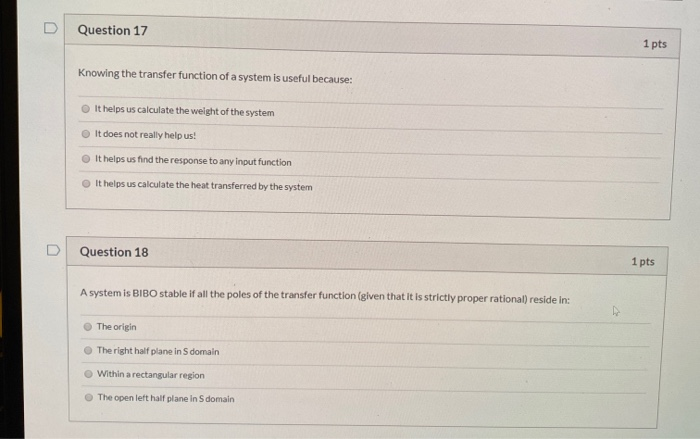Question 17 1 pts Knowing the transfer function of a system is useful because: O It helps us calculate the weight of the system It does not really help us! It helps us find the response to any input function It helps us calculate the heat transferred by the system Question 18 1 pts A system is BIBO stable if all the poles of the transfer function (given that it is strictly proper rational) reside in: The origin The right half plane in s domain Within a rectangular region The open left half plane in S domain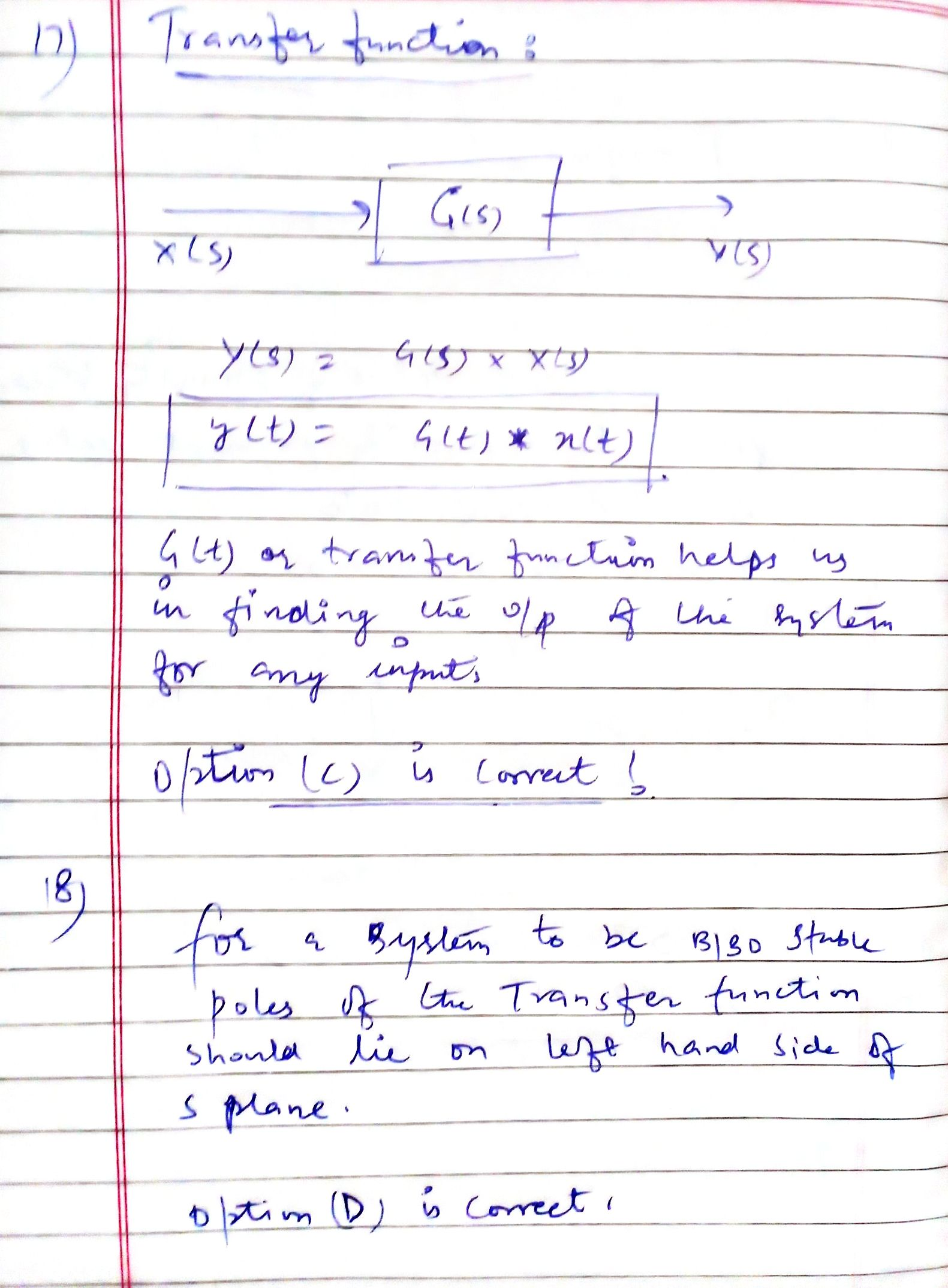##### Add Answer of: Question 17 1 pts Knowing the transfer function of a system is useful because: O It...
Similar Homework Help Questions
• ### D Question 19 1 pts When is a system whose transfer function is an improper rational...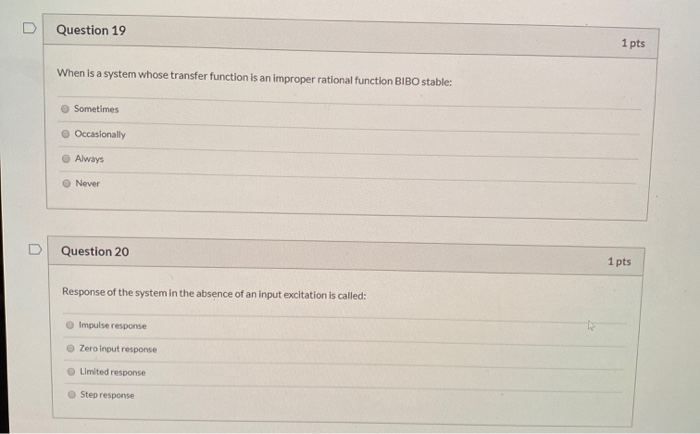D Question 19 1 pts When is a system whose transfer function is an improper rational function BIBO stable: Sometimes Occasionally Always Never Question 20 1 pts Response of the system in the absence of an input excitation is called: Impulse response Zero input response Limited response Step response

• ### A Calculator is allowed. Question 7: The following transfer function is unstable. H(s) = ! Explain...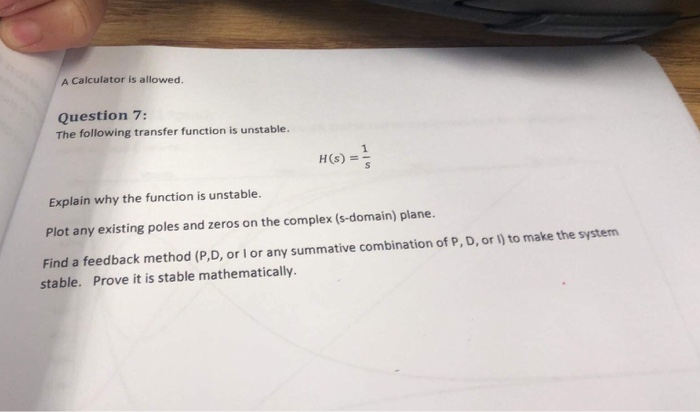A Calculator is allowed. Question 7: The following transfer function is unstable. H(s) = ! Explain why the function is unstable. Plot any existing poles and zeros on the complex (s-domain) plane. Find a feedback method (P,D, or Ior any summative combination of P, D, or I) to make the system stable. Prove it is stable mathematically.

• ### When is a system whose transfer function is an improper rational function BIBO stable: Occasionally Sometimes...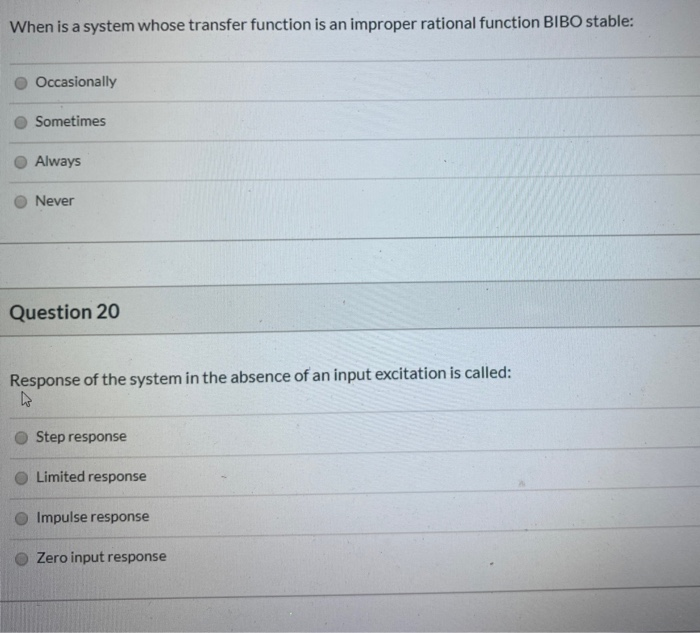When is a system whose transfer function is an improper rational function BIBO stable: Occasionally Sometimes Always Never Question 20 Response of the system in the absence of an input excitation is called: Step response Limited response Impulse response Zero input response

• ### Question 11 1 pts An LTI system is BIBO stable if and only if the impulse...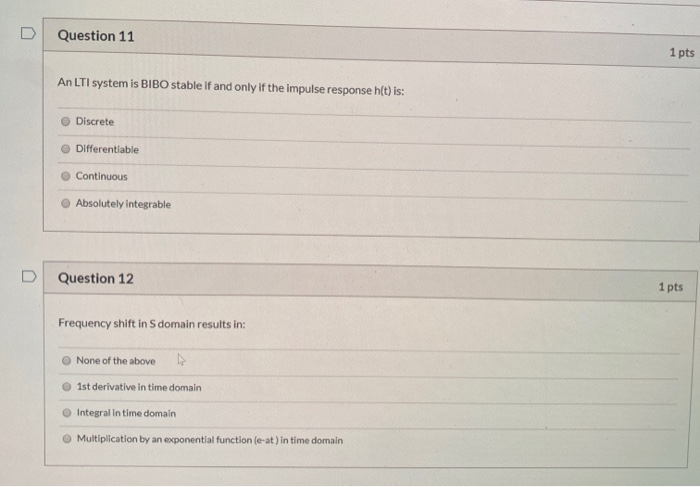Question 11 1 pts An LTI system is BIBO stable if and only if the impulse response h(t) is: Discrete Differentiable Continuous Absolutely integrable Question 12 1 pts Frequency shift in S domain results in: None of the above Ist derivative in time domain Integral in time domain Multiplication by an exponential function (e-at) in time domain

• ### O 0.5 Question 10 1 pts Consider a continuous-time linear system with transfer function (). To...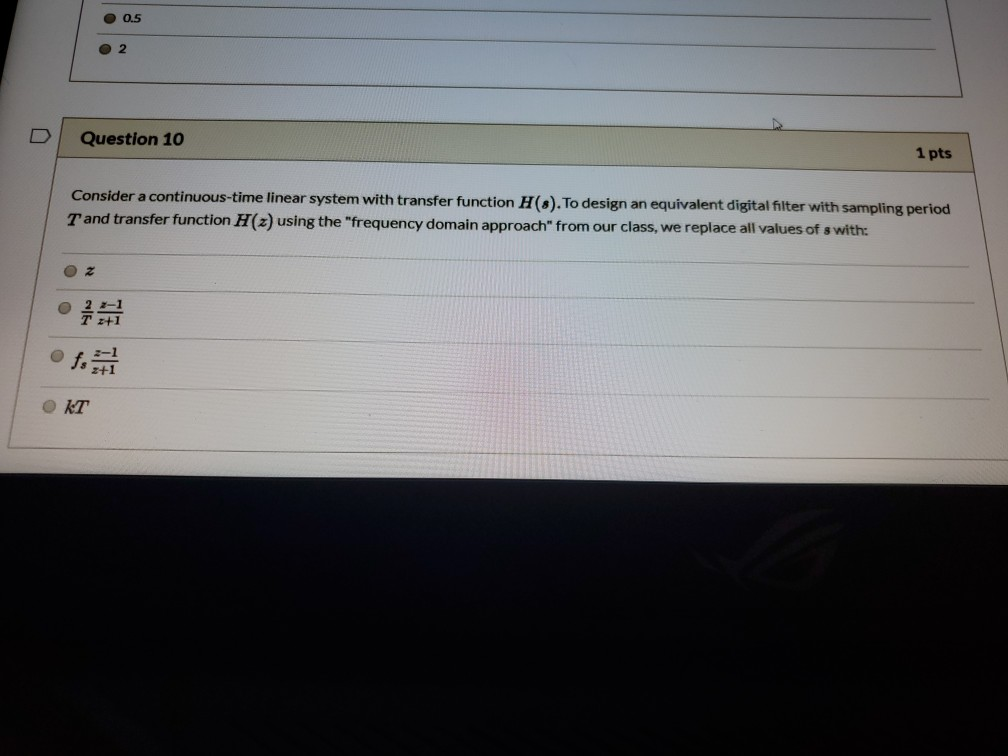O 0.5 Question 10 1 pts Consider a continuous-time linear system with transfer function (). To design an equivalent digital filter with sampling period T and transfer function H(z) using the "frequency domain approach" from our class, we replace all values of s with: Oz OKT

• ### PROBLEM 1 Consider the transfer function T(S) =s5 +2s4 + 2s3 + 4s2 + s + 2 a) Using the Routh-Hurwitz method, determine whether the system is stable. If it is not stable, how many poles are in the...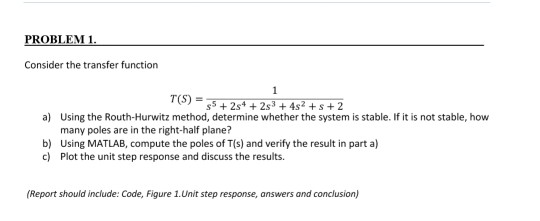PROBLEM 1 Consider the transfer function T(S) =s5 +2s4 + 2s3 + 4s2 + s + 2 a) Using the Routh-Hurwitz method, determine whether the system is stable. If it is not stable, how many poles are in the right-half plane? b) Using MATLAB, compute the poles of T(s) and verify the result in part a) c) Plot the unit step response and discuss the results. (Report should include: Code, Figure 1.Unit step response, answers and conclusion) PROBLEM 1 Consider...

• ### Question# 1 (25 points) For a unity feedback system with open loop transfer function K(s+10)(s+20) (s+30)(s2-20s+2...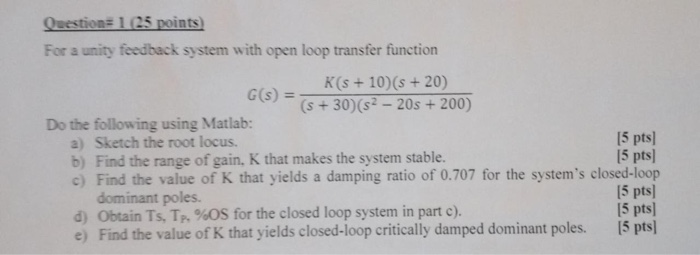Question# 1 (25 points) For a unity feedback system with open loop transfer function K(s+10)(s+20) (s+30)(s2-20s+200) G(s) = Do the following using Matlab: a) Sketch the root locus. b) Find the range of gain, K that makes the system stable c) Find the value of K that yields a damping ratio of 0.707 for the system's closed-loop dominant poles. d) Obtain Ts, Tp, %OS for the closed loop system in part c). e) Find the value of K that yields...

• ### U Question 25 1 pts The difference between an open loop system and a closed loop...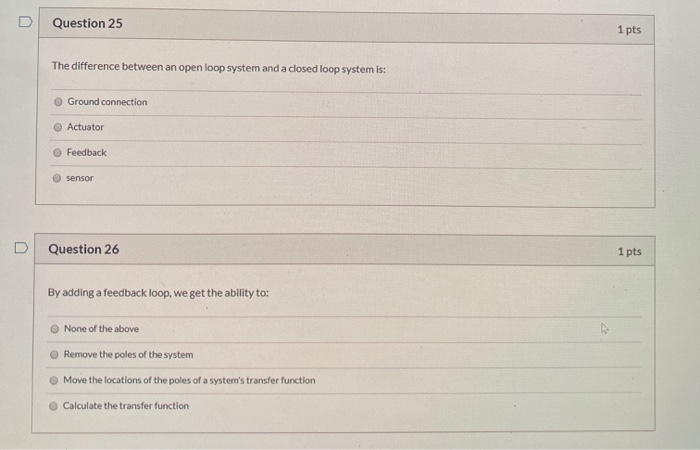U Question 25 1 pts The difference between an open loop system and a closed loop system is: Ground connection Actuator Feedback sensor Question 26 1 pts By adding a feedback loop, we get the ability to: None of the above Remove the poles of the system Move the locations of the poles of a system's transfer function Calculate the transfer function

• ### 2. The Nyquist diagram of a system's loop transfer function is shown in Figure 2. Assume...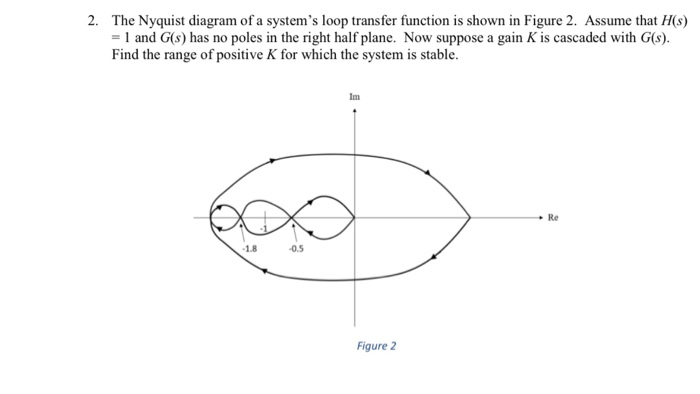2. The Nyquist diagram of a system's loop transfer function is shown in Figure 2. Assume that H(s) 1 and G(s) has no poles in the right half plane. Now suppose a gain K is cascaded with G(s) Find the range of positive K for which the system is stable. Im Re 18 0.5 Figure 2

• ### a = 3 signals and systems 1) [10 pts. Let a system be defined as ta...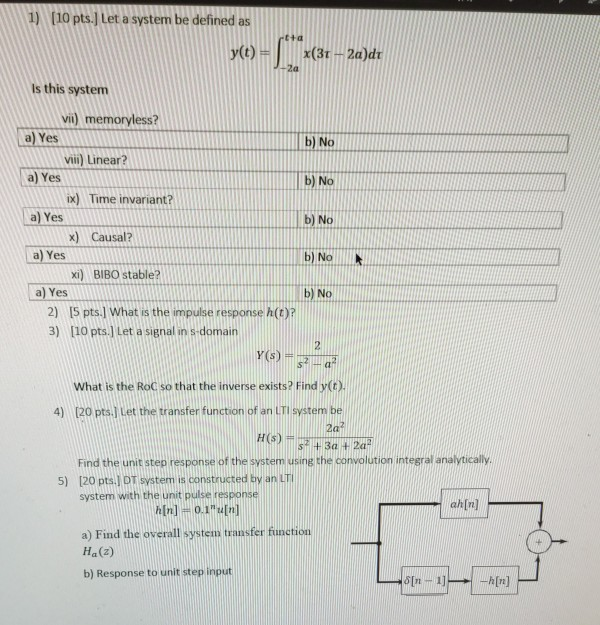a = 3 signals and systems 1) [10 pts. Let a system be defined as ta y(t) x(31 - 2a)dt 2a Is this system b) No b) No b) No vii) memoryless? a) Yes viii) Linear? a) Yes ix) Time invariant? a) Yes x) Causal? a) Yes xi) BIBO stable? a) Yes 2) [5 pts. What is the impulse response h(t)? 3) [10 pts.] Let a signal in s domain b) No b) No 2 Y(S) Sa What is the...

Free Homework App